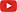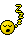## Die don’t remember the last roll. Really. [General Sta­tis­tics]

Hi ElMaestro,

» If it did remember the last roll and the outcome was somehow a function of it, wouldn't that be correlation?

Well, the die isThick As A Brick. If a roll depends on the result of the last one, that could be (a) cheating by an expert gambler or (b) an imperfect die. In both cases you would have a correlation indeed and the concept collapses.

» And how is the die and last roll mental image fitting in with Z and s being indepedent? I think it fits better into the mental picture of errors in a model being IID.

My example deals with $$\small{\mathcal{N}(\mu,\sigma^2)}$$. If you want to dive into combinatorics/permutations (coin flipping, rolling dice) see the first example in this post.

» Or more generally:
» From a sample of size N I am deriving two quantities A and B. Under what cicumstances will A and B be independent? A die with Alzheimer does not really shed light on it, does it?

As usual: Know the data generating process. If you cannot be sure that the outcome of B is independent from A you are in deep shit.

» Kindly help me a little more. I do not see the idea of the R code but it executes nicely …
1. Simulate a $$\small{\mathcal{N}(\mu,\sigma^2)}$$ population $$\small{x}$$ with $$\small{\mu=100},$$ and $$\small{\sigma=20}$$.
For $$\small{N=10^5}$$ the trusted Mersenne-Twister gives us $$\small{\hat{\mu}=100.0655,}$$ $$\small{\hat{\sigma}=20.0154}$$.
Draw a histogram of $$\small{x}$$. Overlay the histogram with a normal curve.
2. Draw 30 samples $$\small{x_{s1},x_{s2},\ldots,x_{s30}}$$ of size 20 from $$\small{x}$$.
Estimate $$\small{\bar{x}, {s_{x}}^{2}}$$ of each sample and plot the normal curves with $$\small{\bar{x}\mp s_x}$$.
Get pooled estimates, which acc. to the CLT should approximate the population’s parameters.
3. Compare the outcome with the population.
4. Correlation of samples’ means and variances. As expected, there is none.

» … except errors for idx.m and idx.v.Fuck. Try the edited one.

» Code on this forum rarely provides clarity for me. This has nothing to do with the code, but has everything to do with meSorry. I added more comments than usual.Dif-tor heh smusma 🖖 Довге життя Україна!Helmut SchützThe quality of responses received is directly proportional to the quality of the question asked. 🚮
Science QuotesIng. Helmut Schütz